Related Articles
Architecture and Learning process in neural network
• Difficulty Level : Hard
• Last Updated : 22 Jan, 2021

In order to learn about Backpropagation, we first have to understand the architecture of the neural network and then the learning process in ANN. So, let’s start about knowing the various architectures of the ANN:

#### Architectures of Neural Network:

ANN is a computational system consisting of many interconnected units called artificial neurons. The connection between artificial neurons can transmit a signal from one neuron to another. So, there are multiple possibilities for connecting the neurons based on which the architecture we are going to adopt for a specific solution. Some permutations and combinations are as follows:

• There may be just two layers of neuron in the network – the input and output layer.
• There can be one or more intermediate ‘hidden’ layers of a neuron.
• The neurons may be connected with all neurons in the next layer and so on …..

So let’s start talking about the various possible architectures:

A. Single-layer Feed Forward Network: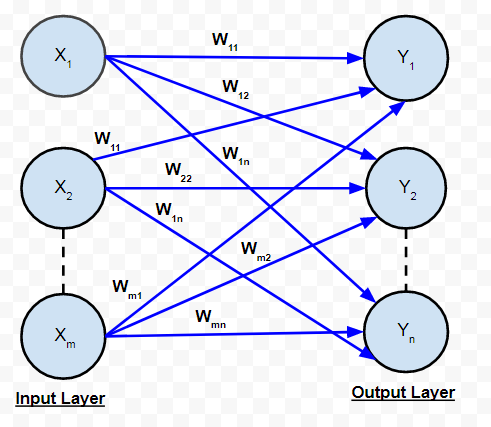It is the simplest and most basic architecture of ANN’s. It consists of only two layers- the input layer and the output layer. The input layer consists of ‘m’ input neurons connected to each of the ‘n’ output neurons. The connections carry weights w11 and so on. The input layer of the neurons doesn’t conduct any processing – they pass the i/p signals to the o/p neurons. The computations are performed in the output layer. So, though it has 2 layers of neurons, only one layer is performing the computation. This is the reason why the network is known as SINGLE layer. Also, the signals always flow from the input layer to the output layer. Hence, the network is known as FEED FORWARD.

The net signal input to the output neurons is given by:The signal output from each output neuron will depend on the activation function used.

B. Multi-layer Feed Forward Network: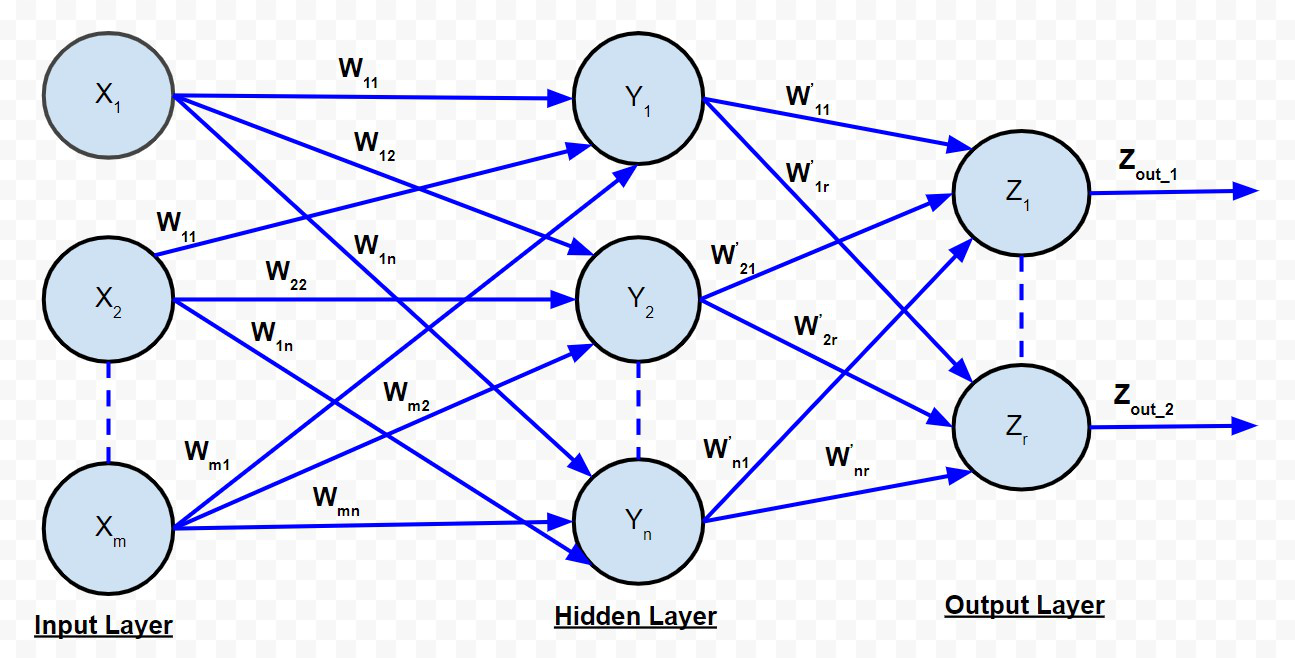Multi-Layer Feed Forward Network

The multi-layer feed-forward network is quite similar to the single-layer feed-forward network, except for the fact that there are one or more intermediate layers of neurons between the input and output layer. Hence, the network is termed as multi-layer. Each of the layers may have a varying number of neurons. For example, the one shown in the above diagram has ‘m’ neurons in the input layer and ‘r’ neurons in the output layer and there is only one hidden layer with ‘n’ neurons.for the kth hidden layer neuron. The net signal input to the neuron in the output layer is given by:C. Competitive Network:

It is as same as the single-layer feed-forward network in structure. The only difference is that the output neurons are connected with each other (either partially or fully). Below is the diagram for this type of network.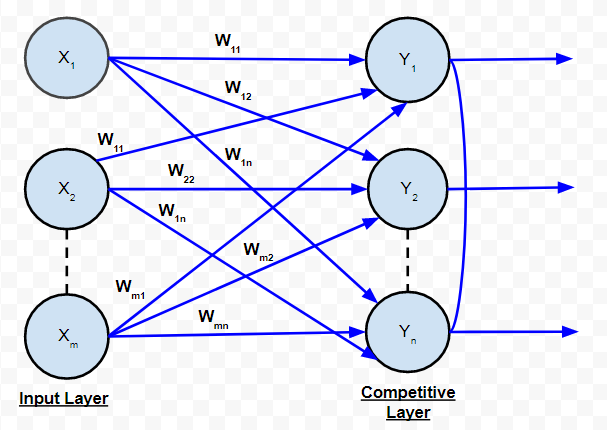Competitive Network

According to the diagram, it is clear that few of the output neurons are interconnected to each other. For a given input, the output neurons compete against themselves to represent the input. It represents a form of an unsupervised learning algorithm in ANN that is suitable to find the clusters in a data set.

D. Recurrent Network: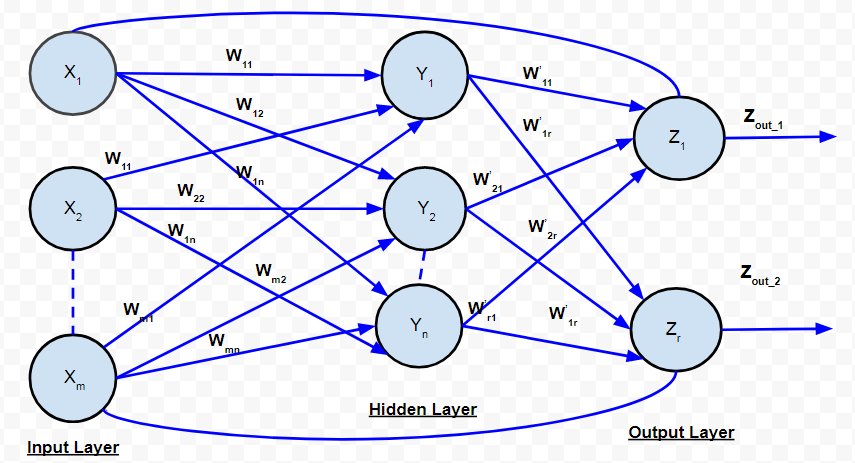Recurrent Network

In feed-forward networks, the signal always flows from the input layer towards the output layer (in one direction only). In the case of recurrent neural networks, there is a feedback loop (from the neurons in the output layer to the input layer neurons). There can be self-loops too.

#### Learning Process In ANN:

Learning process in ANN mainly depends on four factors, they are:

1. The number of layers in the network (Single-layered or multi-layered)
2. Direction of signal flow (Feedforward or recurrent)
3. Number of nodes in layers: The number of node in the input layer is equal to the number of features of the input data set. The number of output nodes will depend on possible outcomes i.e. the number of classes in case of supervised learning. But the number of layers in the hidden layer is to be chosen by the user. A larger number of nodes in the hidden layer, higher the performance but too many nodes may result in overfitting as well as increased computational expense.
4. Weight of Interconnected Nodes: Deciding the value of weights attached with each interconnection between each neuron so that a specific learning problem can be solved correctly is quite a difficult problem by itself. Take an example to understand the problem. Take the example of a Multi-layered Feed-Forward Network, we have to train an ANN model using some data, so that it can classify a new data set, say p_5(3,-2). Say we have deduced that p_1=(5,2)   and  p_2 = (-1,12)   belonging to class C1 while p_3=(3,-5)   and p_4 = (-2,-1)  belonging to class C2. We assume the values of synaptic weights w_0,w_1,w_2 as -2, 1/2 and 1/4 respectively. But we will NOT get these weight values for every learning problem. For solving a learning problem with ANN, we can start with a set of values for synaptic weights and keep changing those in multiple iterations. The stopping criterion may be the rate of misclassification < 1% or the maximum numbers of iterations should be less than 25(a threshold value). There may be another problem that, the rate of misclassification may not reduce progressively.

So, we can summarize the learning process in ANN as the combination of – deciding the number of hidden layers, the number of nodes in each of the hidden layers, the direction of signal flow, deciding the connection weight.

Multi-layer feed network is a commonly used architecture. It has been observed that a neural network with even one hidden layer can be used to reasonably approximate any continuous function. The learning methodology adopted to train a multi-layer feed-forward network is Backpropagation

#### Backpropagation:

In the above section, we get to know that the most critical activities of training an ANN are to assign the inter-neuron connection weights. In 1986, an efficient way of training an ANN was introduced. In this method, the difference in output values of the output layer and the expected values, are propagated back from the output layer to the preceding layers. Hence, the algorithm implementing this method is known as BACK PROPAGATION i.e. propagating the errors back to the preceding layers.

The backpropagation algorithm is applicable for multi-layer feed-forward network. It is a supervised learning algorithm which continues adjusting the weights of the connected neurons with an objective to reduce the deviation of the output signal from the target output. This algorithm consists of multiple iterations, known as epochs. Each epoch consists of two phases:

• Forward Phase: Signal flow from neurons in the input layer to the neurons in the output layer through the hidden layers. The weights of the interconnections and activation functions are used during the flow. In the output layer, the output signals are generated.
• Backward Phase: Signal is compared with the expected value. The computed errors are propagated backwards from the output to the preceding layer. The error propagated back are used to adjust the interconnection weights between the layers.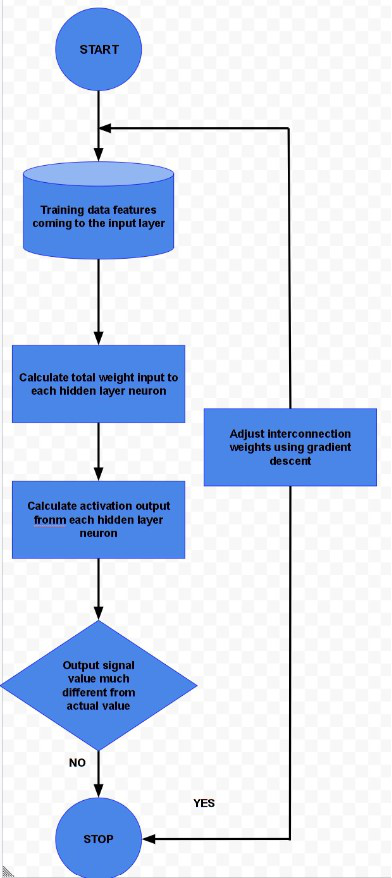BACKPROPAGATION

The above diagram depicts a reasonably simplified version of the back propagation algorithm.

One main part of the algorithm is adjusting the interconnection weights. This is done using a technique termed as Gradient Descent. In simple words, the algorithm calculates the partial derivative of the activation function by each interconnection weight to identify the ‘gradient’ or extent of change of the weight required to minimize the cost function.

In order to understand the back propagation algorithm in detail, let us consider the Multi-layer Feed Forward Network.The net signal input to the hidden layer neurons is given by:Ifis the activation function of the hidden layer, thenThe net signal input to the output layer neurons is given by: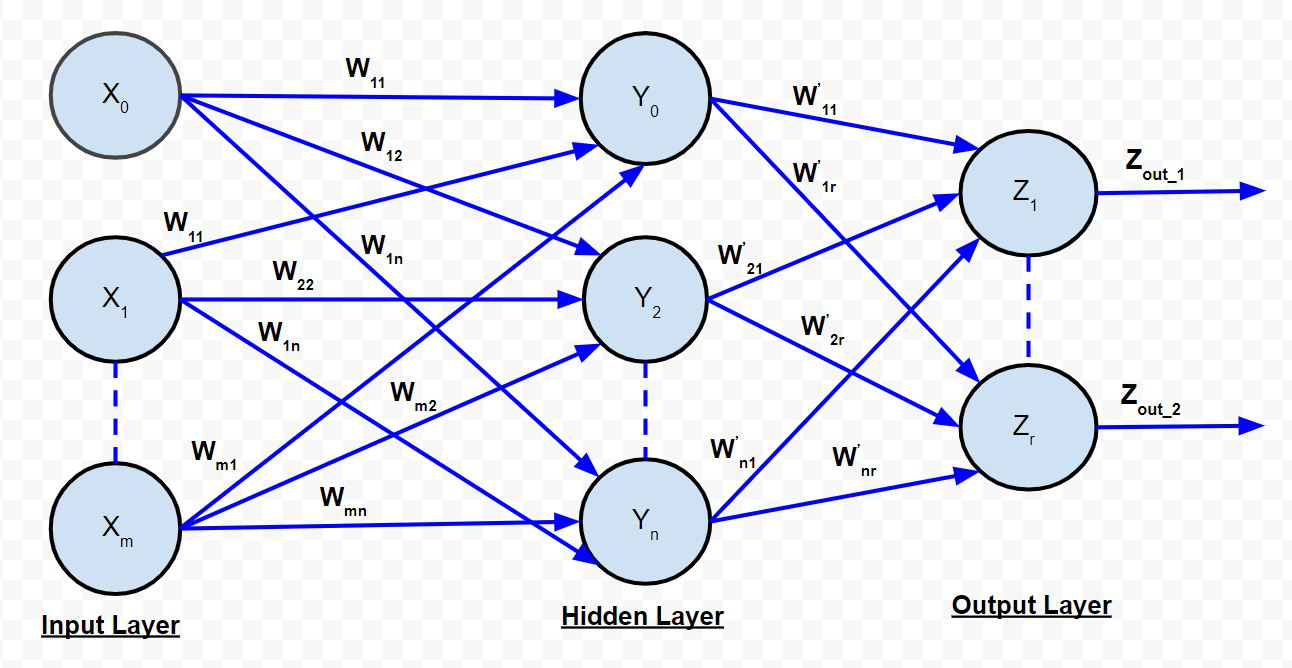BACKPROPAGATION NET

Note that the signalsandare assumed to be 1. Ifis the activation function of the hidden layer, thenIf is the target of the k-th output neuron, then the cost function defined as the squared error of the output layer is given by:According to the descent algorithm, partial derivative of cost function E has to be taken with respect to interconnection weights. Mathematically it can be represented as:{Above expression is for the interconnection weights between the j-th neuron in the hidden layer and the k-th neuron in the output layer.} This expression can be reduced towhere,orIf we assumeas a component of the weight adjustment needed for weightcorresponding to the k-th output neuron, then :On the basis of this, the weights and bias need to be updated as follows:

• For weights:• Hence,• For bias:• Hence,In the above expressions, alpha is the learning rate of the neural network. Learning rate is a user parameter which decreases or increases the speed with which the interconnection weights of a neural network is to be adjusted. If the learning rate is too high, the adjustment done as a part of the gradient descent process may diverge the data set rather than converging it. On the other hand, if the learning rate is too low, the optimization may consume more time because of the small steps towards the minima.

{All the above calculations are for the interconnection weight between neurons in the hidden layer and neurons in the output layer}

Like the above expressions, we can deduce the expressions for “Interconnection weights between the input and hidden layers:

• For weights:• Hence,• For bias:• Hence,So, in this way, we can use the Backpropagation algorithm to solve various Artificial Neural Networks.My Personal Notes arrow_drop_up